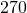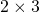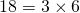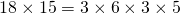# Highest Common Factor of small integers

Let’s list all the factors of 12 and of 8:

Factors of 12 are: 1, 2, 3, 4, 6, 12

Factors of 8 are: 1, 2, 4, 8

By examining the lists, the highest factor common to both integers 4.

This kind of search is known as an exhaustive search – we list all the factors so all possible values are considered. For small numbers, this is a fast approach especially if it can be done mentally. For large numbers, it can be better to use the prime factor method.

# Lowest Common Multiple of small integers

Let’s find the lowest common multiple of 15 and 18.

Of course, the numberwhich isis a common multiple of 15 and of 18. However, it is not the lowest common multiple.

Multiples of 15 are: 15, 30, 45, 60, 75, 90, 105, 120, …

Multiples of 18 are: 18, 36, 54, 72, 90, good, we can stop here.

The first multiple that we come to that is common to both is 90.

This method is good when the numbers are relatively small.

# Skill 2a

Practice finding the HCF and the LCM by recalling multiplication tables.

# Highest Common Factor using the prime factor method

Example 1: Let’s find the HCF of 24 and 30 using the prime factor method.Prime factors common to both 24 and 30 are:. Thereforeis the HCF of 24 and 30.

Example 2: Let’s see how this works with much larger values:Common to both:That is, HCF of 360 and 400 is.

Notice that;. The numbersandare known as coprime – they don’t have any common factors.

# Lowest Common Multiple Formula

Find the LCM of 15 and 18.

Now,Note that:Therefore,Notice that 3, the HCF, is repeated when we multiply.

Because we used 3, the HCF, the divisors 5 and 6 share no common factor.

We can be sure thatis a common multiple (it isand). We can also be sure that it is the lowest common multiple as 5 and 6 do not share any factor that could be removed.Therefore# Skill 2b

Use the prime factor method to find the HCF of the two numbers given. Then use the formula or otherwise to find the LCM.